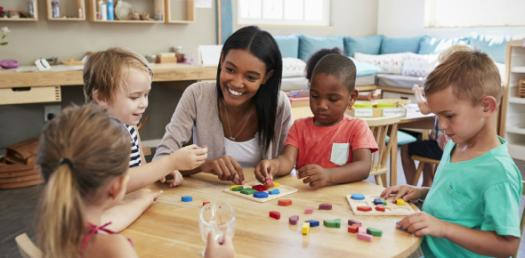# Kindergarten Level Basic Addition Test! Quiz

Approved & Edited by ProProfs Editorial Team
At ProProfs Quizzes, our dedicated in-house team of experts takes pride in their work. With a sharp eye for detail, they meticulously review each quiz. This ensures that every quiz, taken by over 100 million users, meets our standards of accuracy, clarity, and engagement.
| Written by Stacy1km
S
Stacy1km
Community Contributor
Quizzes Created: 1 | Total Attempts: 436
Questions: 5 | Attempts: 439SettingsAre you looking for the Kindergarten Level Basic Addition Test? If you have been having a hard time when it comes to solving basic math problems regarding the addition function, you are in luck as the quiz below is the answer to the how to be better. All you need to do is give it a try and answer.

• 1.

### 1+2 =

• A.

4

• B.

3

• C.

2

• D.

45

B. 3
Explanation
The correct answer is 3 because when you add 1 and 2 together, the sum is 3.

Rate this question:

• 2.

• A.

True

• B.

False

B. False
• 3.

1
• 4.

### 2+2= 4

• A.

True

• B.

False

A. True
Explanation
The given equation "2+2=4" is a basic addition problem where adding 2 and 2 results in 4. Therefore, the correct answer is "True" as the equation is mathematically accurate.

Rate this question:

• 5.

### 3+3 =

• A.

1

• B.

4

• C.

6

• D.

65Back to top